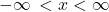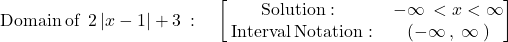## Select the correct answer. What is the domain of f(x) = 2|x − 1| + 3? A. {x | x ≥ 3} B. {x | x < 1}

Question

What is the domain of f(x) = 2|x − 1| + 3?

A.
{x | x ≥ 3}
B.
{x | x < 1}
C.
{x | x ≤ -1}
D.
{x | x = all real numbers}

in progress 0
4 weeks 2021-08-18T03:48:47+00:00 1 Answers 0 views 0

## {x | x = all real numbers}

Step-by-step explanation:

The domain of a function is the set of input or argument values for which the function is real and defined.

The function has no undefined points nor domain constraints. Therefore, the domain is